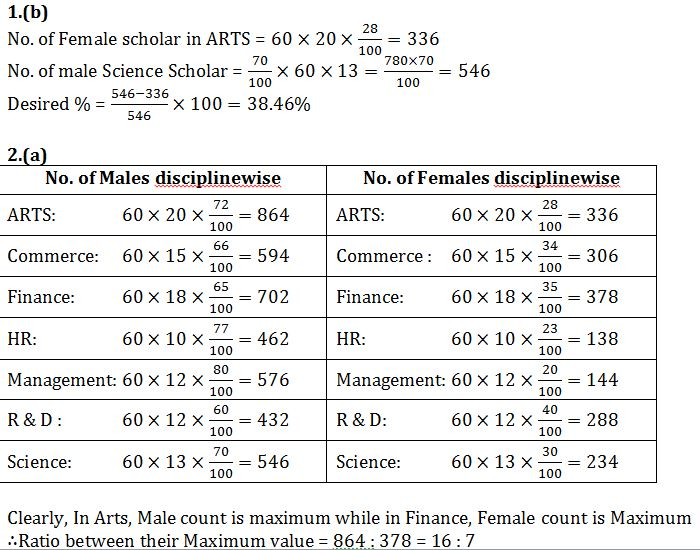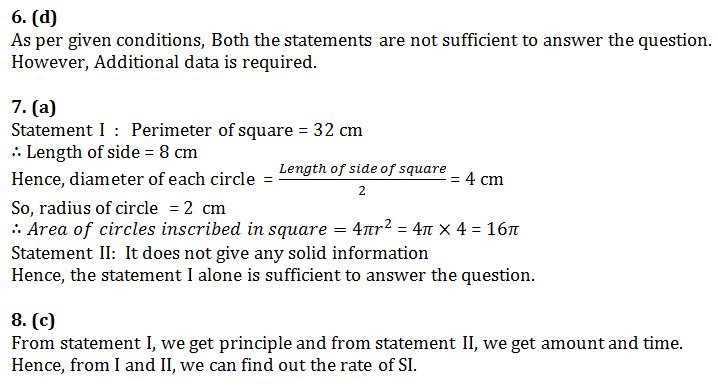# Quant Quiz (DI, DS) with detailed solution 5th September 2018

Directions (1 to 5): The pie chart given below shows the percentage of Scholars in different disciplines in California Uninversity for the Year 2016 and The table suggests the percentage of girls.Percentage of Girls in Disciplines

 Disciplines % of Girls Arts 28 Commerce 34 Finance 35 HR 23 Management 20 R&D 40 Science 30

Q1. Number of girls scholar in Arts discipline is what percent more or less than the no. of Male Scholar in Science?

(a)39.46%

(b)38.46%

(c)40.5%

(d)42.82%

(e)None of the above

Q2. What is the ratio of Maximum number of Boys in a discipline given to the maximum no. of girls in a discipline?

(a)16 : 7

(b)16 : 9

(c) 15 : 7

(d)7 : 16

(e)None of these

Q3. What is the difference between the average number of boys in all disciplines together to the average number of girls in all disciplines together(approximately)?

(a)222

(b)289

(c)300

(d)336

(e)312

Q4. Total number of scholars in Commerce, Arts and Science is what percent of Total number of Male scholar in R&D, Management and Finance together?

(a) 152.76%

(b) 158.48%

(c) 168.42%

(d) 178 %

(e)None of the above

Q5. Due to some natural calamities in USA, 80 % of the scholars from Management left the University . Approximately, What would be the new central angle of Commerce scholar as per current availability? ( However, no students from other discipline left the University). (Approximately)

(a)85

(b)70

(c) 75

(d)55

(e)60

Directions (6 to 10): Each of the questions given below is followed by two statements numbered I and II.You have to decide whether the data provided in the statements are sufficient to answer the questions from the following options.

(a). If the statement I alone is sufficient to provide the answer.

(b). If the statement II alone is sufficient to provide the answer.

(c).  If both statement are required to provide the answer.

(d). If neither statement I nor II is sufficient to provide the answer.

(e).  If either statement I or II is sufficient to provide the answer.

Q6. What is the cost of painting a room which is of the form of a cube?

I. The base area of the room is 144 sq. ft

II. The room has one door of size 6’ x 4’ and has no window

Q7. Four circles of equal radii are inscribed in a square touching each other. What is the area covered by the four circles?

I. The perimeter of the square is 32 cm.

II.The ratio of sums of areas of four circles  to that of the square is 3:4.

Q8. What percentage rate of simple interest per annum did Ashok pay to Sudhir?

I. Ashok borrowed Rs. 8800 from Sudhir

II.Ashok returned Rs. 10000 to Sudhir at the end of 2 year and settled the loan.

Q9. A toy was initially listed at a price to give the shopkeeper 20% profit of the wholesale cost. What is the wholesale cost?

I. After reducing the listed price by 10%, the toy sold for a profit of Rs. 19

II.The toy sold for Rs. 60.

Q10. Amongst the five students A, B, C, D and E who got the maximum marks?

I. D got more marks than A and C.

II.B got less than E but more than D.

## Solution